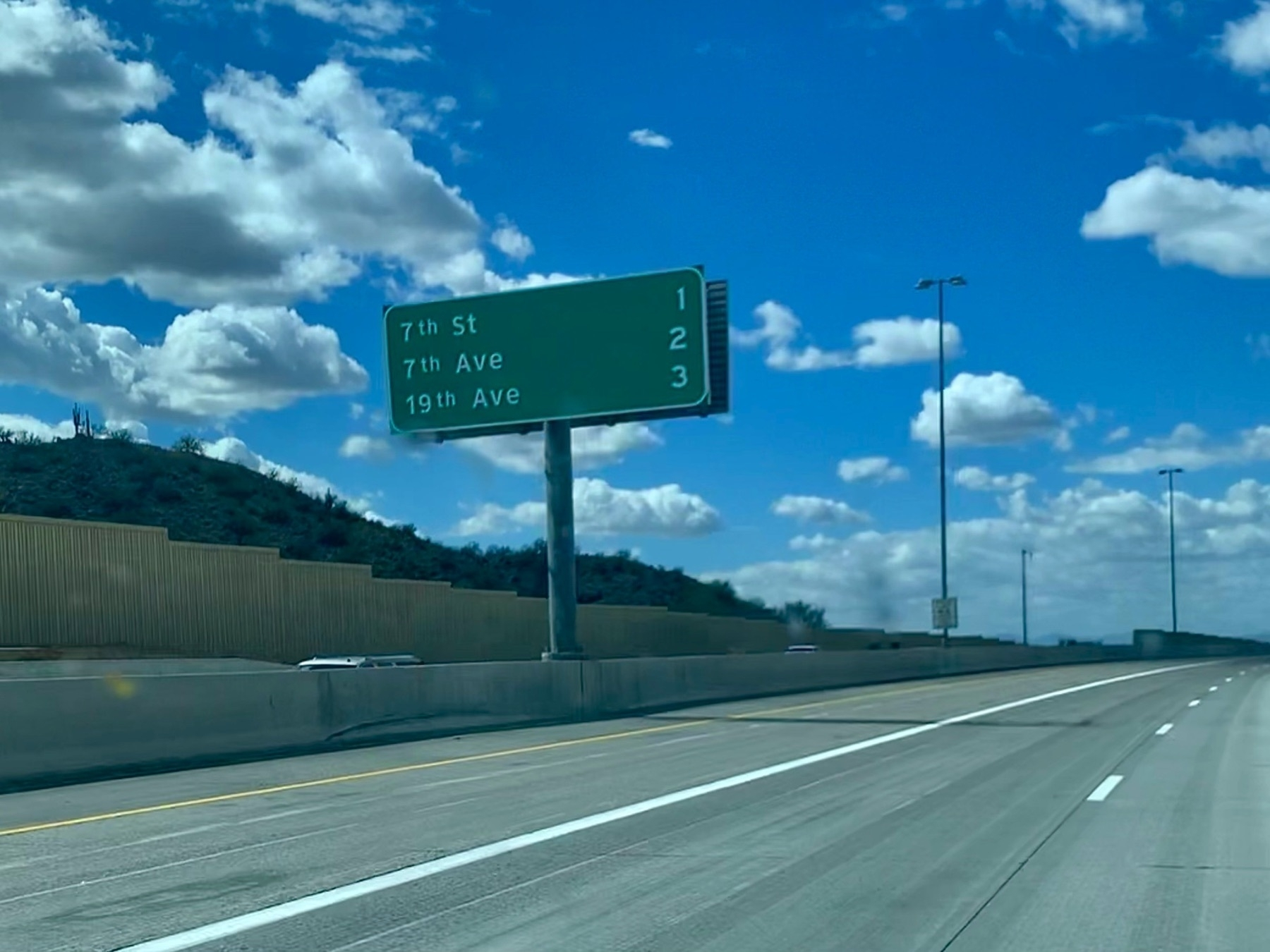## Saratoga

Simple math on the way to Saratoga.

$$14 + 10 = 24$$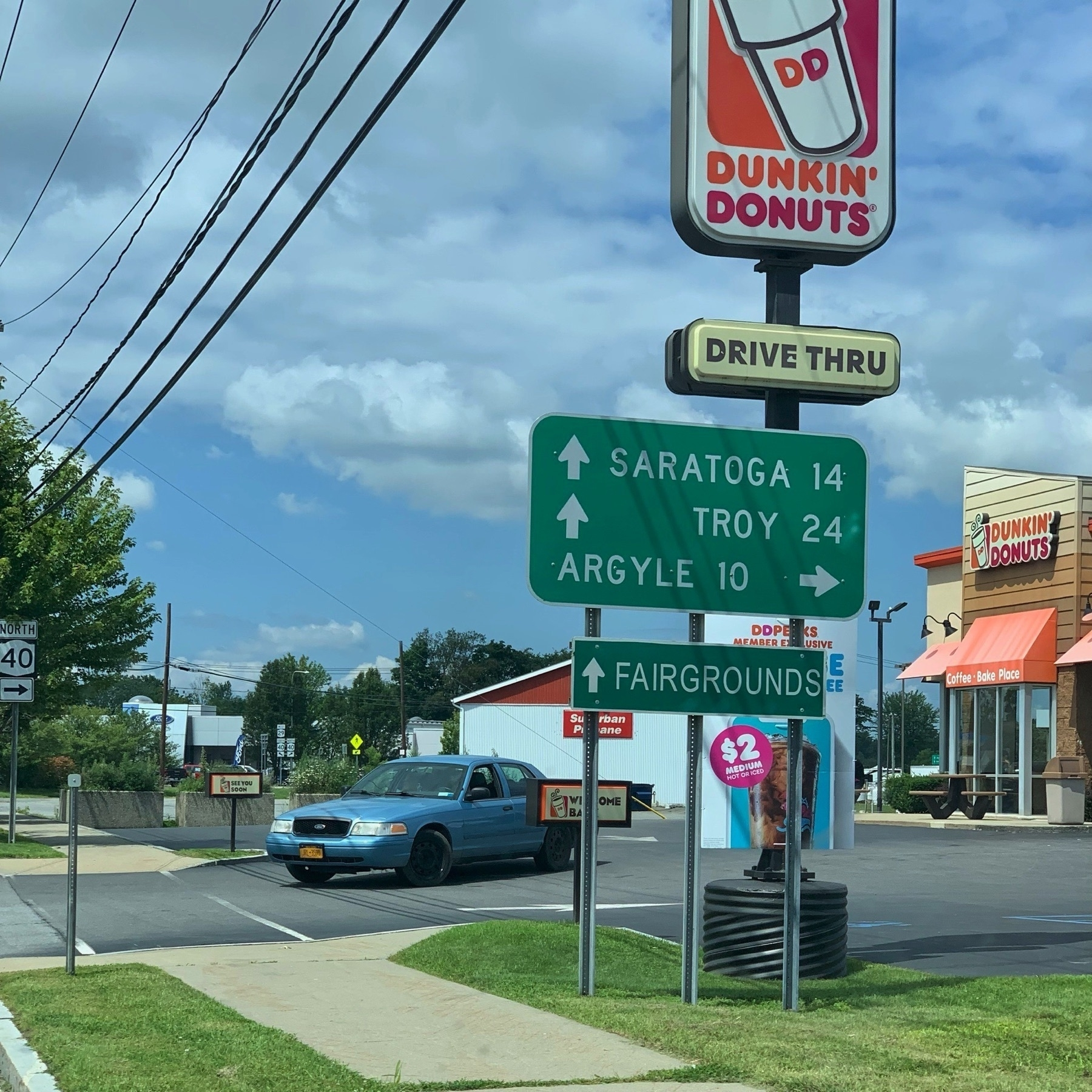## Greenbush

$$9 + 11 = 20$$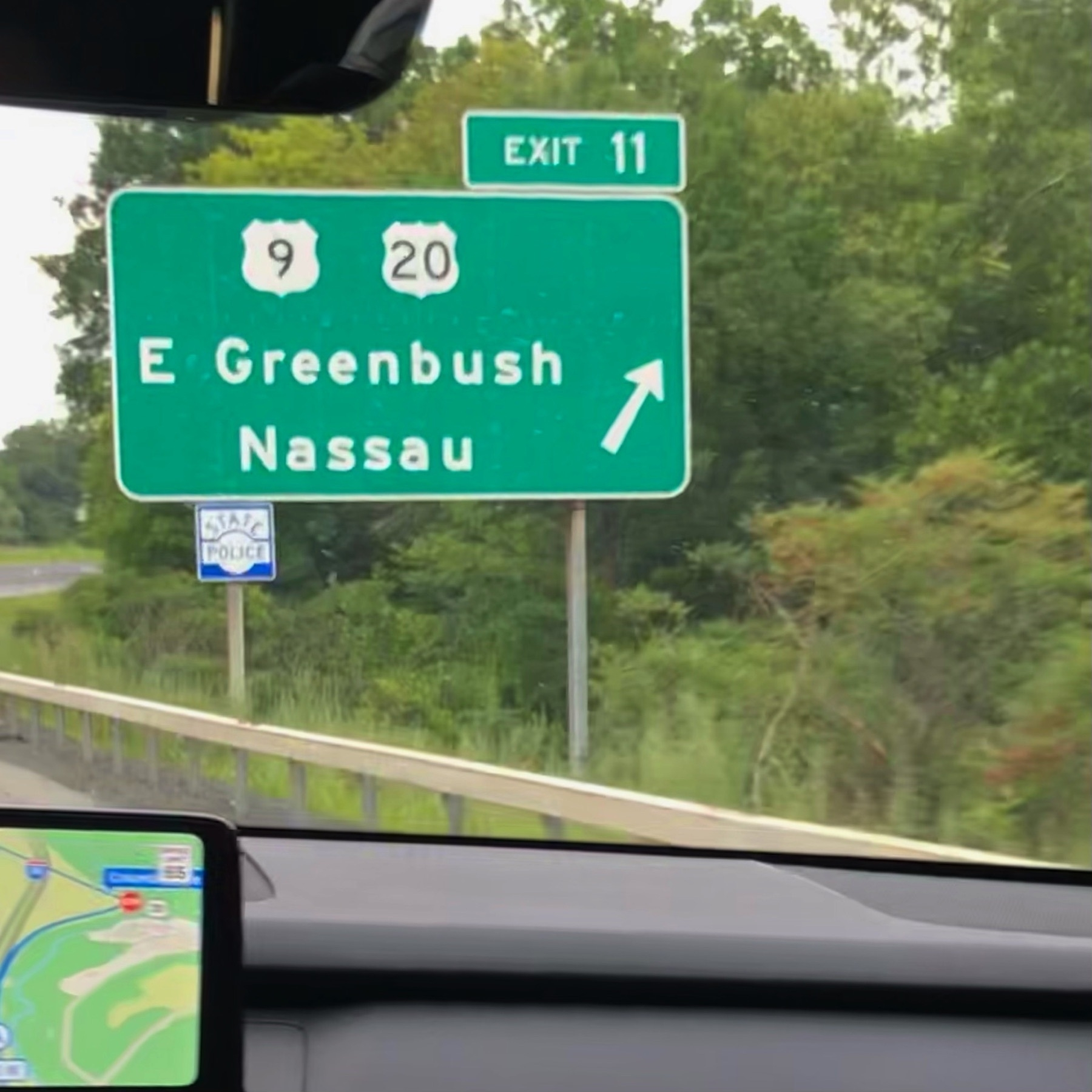## Wurtsboro

We found this sign while driving back from visiting the Woodstock site and driving back to Accord, NY.

$$12 + 1 = 13$$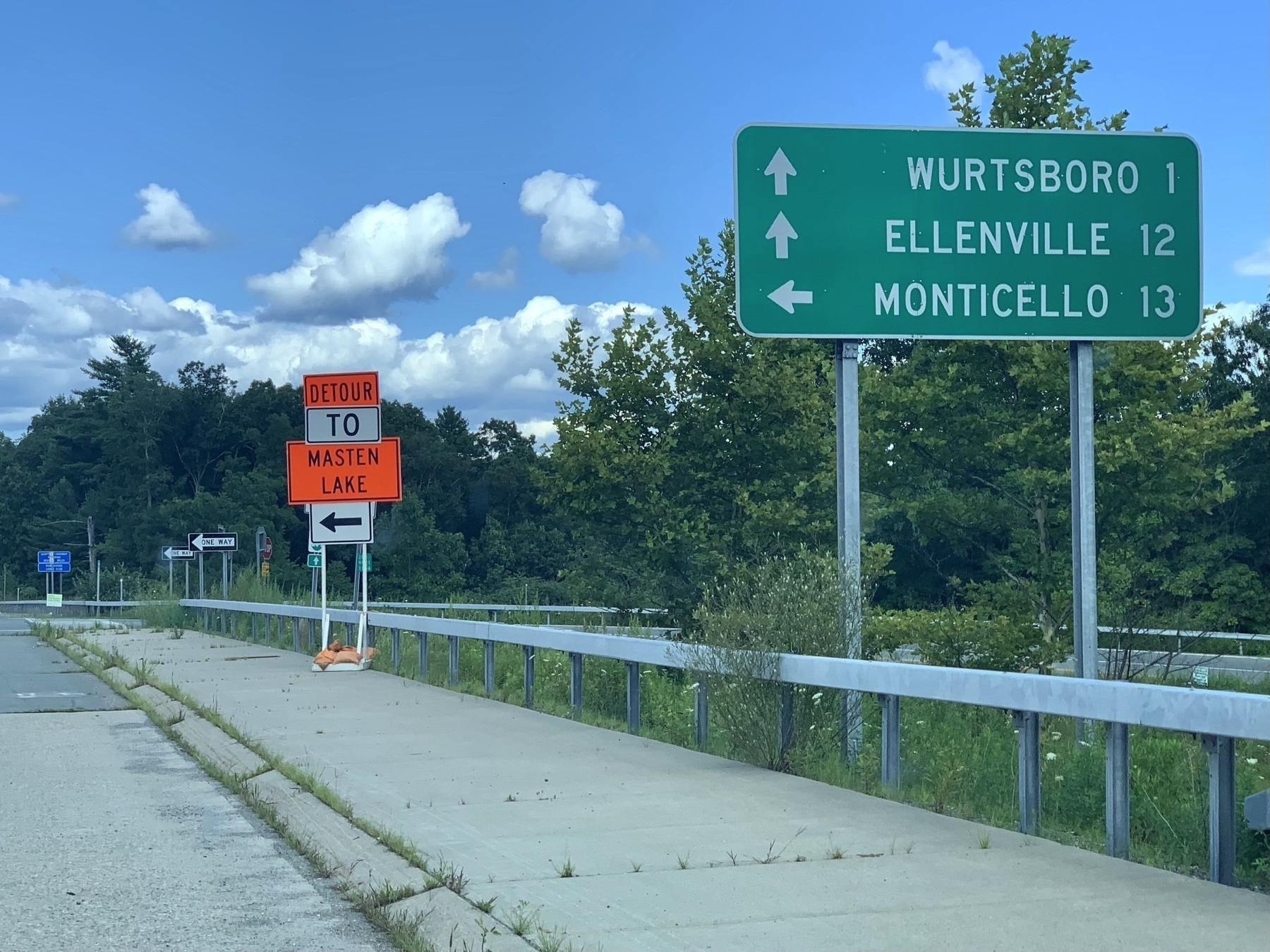## Gross Vehicle Weight

Some fun math along I-80/I-90.

$$( 70 \div ( 90 - 80 ) ) + 13 = 65 - 45$$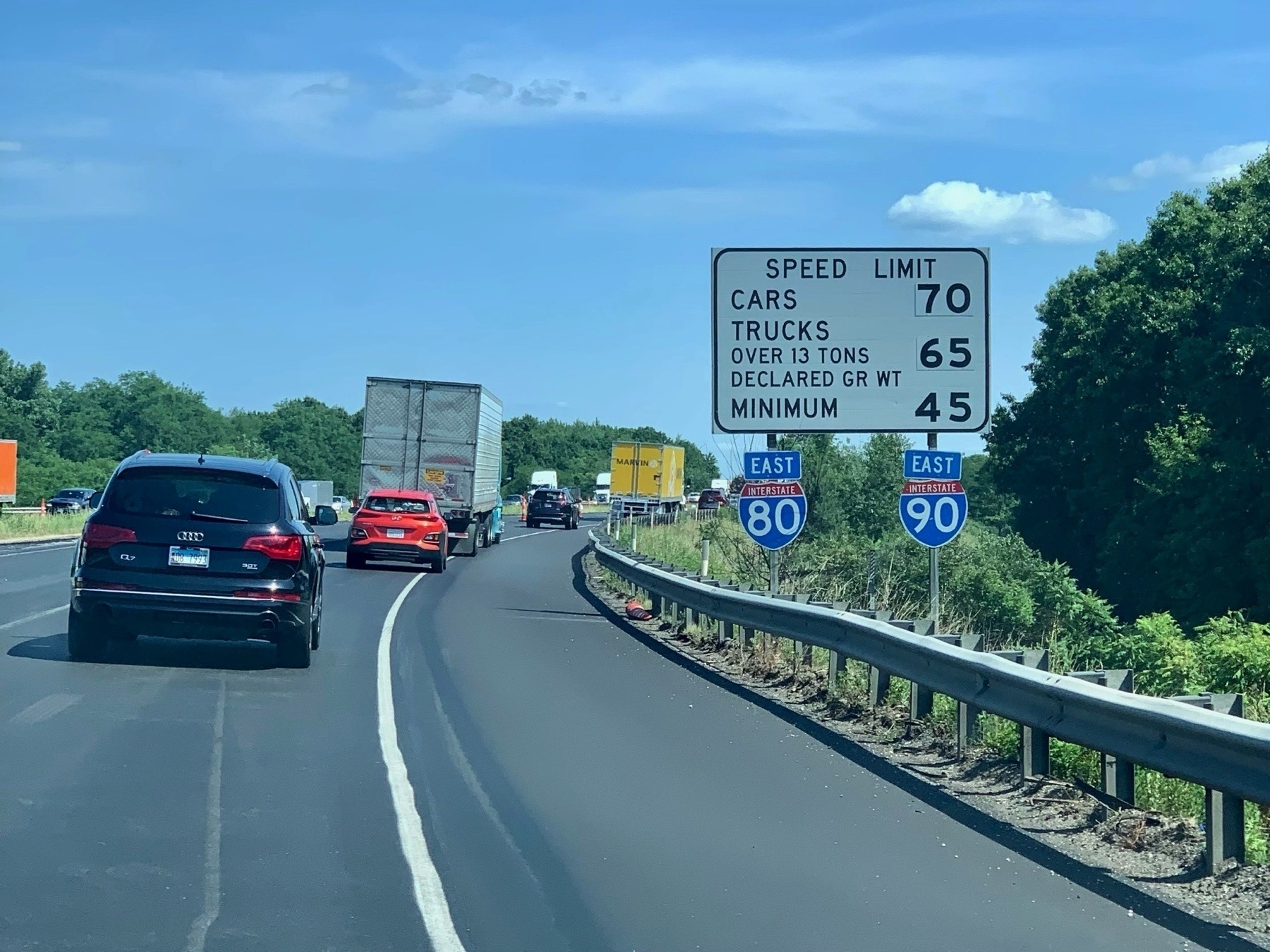## Phoenix Math

Tammy Thingelstad found this amazing Road Sign Math winner on Highway 101 in Phoenix, Arizona! This is the first winning sign found in years to reboot the great game of finding math equations in road signs!

$$7 + 7 + ( 3 \times 2 ) = 19 + 1$$